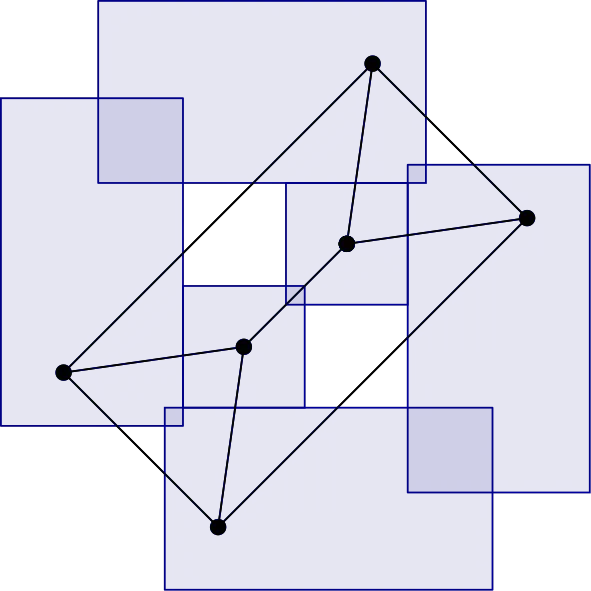# Exploring Boxicity: Graph Representations in Multidimensional Spaces## Problem Statement

Boxicity, a graph parameter introduced by Roberts (1969), has emerged as a tool for measuring the complexity of social or ecological networks. Given a graph $G$, its boxicity is the smallest dimension $d$ in which it can be represented as the intersection graph of hyperboxes in $\mathbb{R}^d$. This means that there exists a bijection between the graph’s vertices and the hyperboxes, wherein two vertices are adjacent if and only if the corresponding hyperboxes intersect. Researchers have diligently explored this parameter discovering intriguing connections with other well-established graph parameters like tree width and chromatic number, and deriving bounds for multiple graph classes such as bipartite and planar graphs.

During the initial exploration of boxicity, Cozzens and Roberts (1983) presented a fruitful redefinition of boxicity in terms of covering graph edges with co-interval graphs. This method found successful application in subsequent research leading to new lower bounds on boxicity. Recently, Caoduro and Sebő (2023) further refined this approach to a completely combinatorial methodology for obtaining bounds on the boxicity of Kneser graphs, line graphs, and co-line graphs. The potential of these methodologies invites further study, promising to yield bounds for several other graph classes and allowing the investigation of related conjectures such as Wegner’s conjecture (1965).

In this project, students will have the occasion to strengthen their knowledge of graph theory and delve into geometric techniques for graph representation. Beginning with a review of relevant literature on boxicity, participants will apply different methodologies to establish bounds for boxicity across various graph classes. In addition, students will be tasked with developing heuristics and algorithms to ascertain the boxicity of select graphs from the studied graph classes. This pivotal step often yields critical insights and intuitions regarding the behavior of the respective graph classes.

### Details

• Expected team size: 3
• Student Experience Level: Intermediate: students who have an introduction to proofs
##### Prerequisites
• Graph Theory
• Introduction to Discrete Mathematics
##### Skills
• Python
• $\LaTeX$